Study Materials

# NCERT Solutions for Class 12th Mathematics-II

Page 5 of 12

## Chapter 7. Integrals

### Exercise 7.5 (3)

Exercise 7.5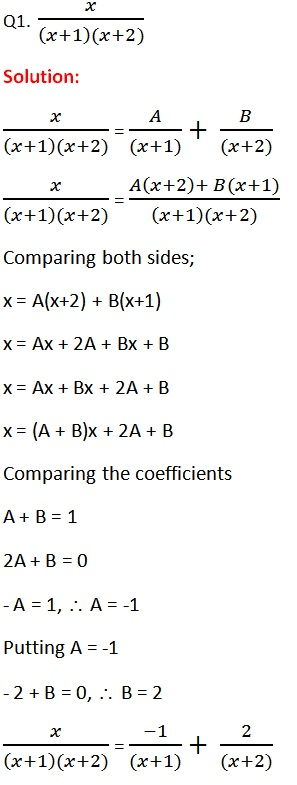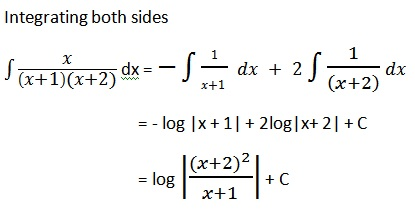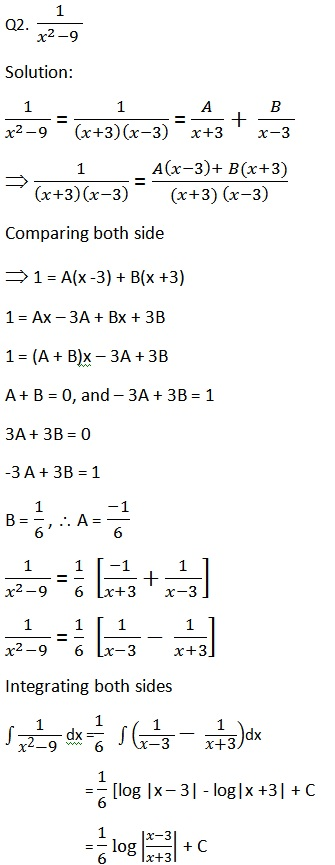Q3.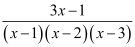Solution: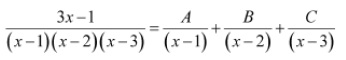3x -1 = A(x-2)(x-3) + B(x-1)(x-3) + C(x-1)(x-2)  ................... (i)

Substituting x =1, 2, and 3 respectively in equation (i), we obtain A =1, B = -5 and C= 4.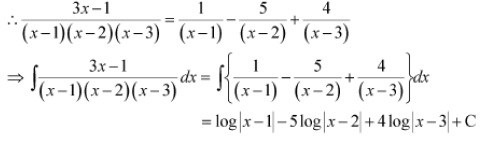Q4.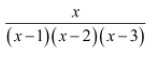Solution: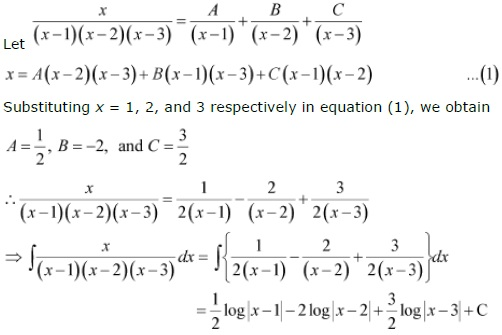Q5.Solution: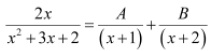2x = A(x + 2) + B(x +1)

Substituting x = −1 and −2 in equation (1), we obtain
A = −2 and B = 4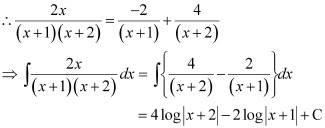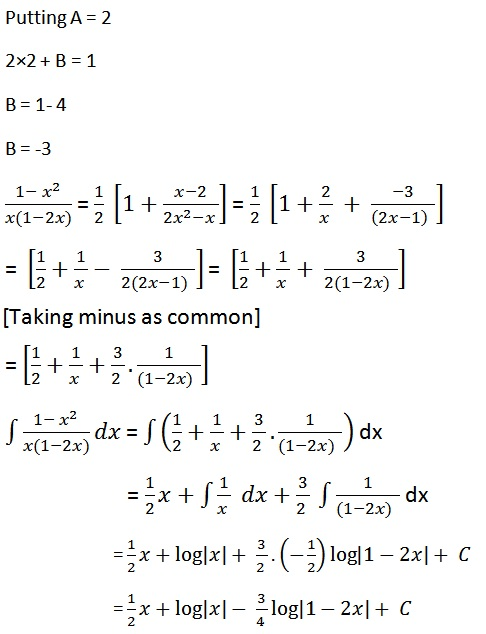Page 5 of 12

Chapter Contents: